# kalman滤波原理（通俗易懂）

#### 2．卡尔曼滤波器的介绍

（Introduction to the Kalman Filter）

#### 3． 卡尔曼滤波器算法

（The Kalman Filter Algorithm）

X(k)=A X(k-1)+B U(k)+W(k)

Z(k)=H X(k)+V(k)

X(k|k-1)=A X(k-1|k-1)+B U(k) ……….. (1)

P(k|k-1)=A P(k-1|k-1) A’+Q ……… (2)

X(k|k)= X(k|k-1)+Kg(k) (Z(k)-H X(k|k-1)) ……… (3)

Kg(k)= P(k|k-1) H’ / (H P(k|k-1) H’ + R) ……… (4)

P(k|k)=（I-Kg(k) H）P(k|k-1) ……… (5)

#### 4． 简单例子

（A Simple Example）

X(k|k-1)=X(k-1|k-1) ……….. (6)

P(k|k-1)=P(k-1|k-1) +Q ……… (7)

X(k|k)= X(k|k-1)+Kg(k) (Z(k)-X(k|k-1)) ……… (8)
Kg(k)= P(k|k-1) / (P(k|k-1) + R) ……… (9)
P(k|k)=（1-Kg(k)）P(k|k-1) ……… (10)

××××××××××××××××××

#### 附matlab下面的kalman滤波程序：

clear
N=200;
w(1)=0;
w=randn(1,N)
x(1)=0;
a=1;
for k=2:N;
x(k)=a*x(k-1)+w(k-1);
end

V=randn(1,N);
q1=std(V);
Rvv=q1.^2;
q2=std(x);
Rxx=q2.^2;
q3=std(w);
Rww=q3.^2;
c=0.2;
Y=c*x+V;

p(1)=0;
s(1)=0;
for t=2:N;
p1(t)=a.^2*p(t-1)+Rww;
b(t)=c*p1(t)/(c.^2*p1(t)+Rvv);
s(t)=a*s(t-1)+b(t)*(Y(t)-a*c*s(t-1));
p(t)=p1(t)-c*b(t)*p1(t);
end

t=1:N;
plot(t,s,'r',t,Y,'g',t,x,'b');# 卡尔曼滤波 -- 从推导到应用(一)

卡尔曼滤波器是在估计线性系统状态的过程中，以最小均方误差为目的而推导出的几个递推数学等式,也可以从贝叶斯推断的角度来推导。

本文将分为两部分：

“很简单.” A说,于是他丝毫不差地沿着小径走到了树下.

“也不难，我当过特种兵。” B说，于是他歪歪扭扭地走到了树旁。“唉，好久不练，生疏了。” （只凭自己的预测能力）

“看我的，我有 DIY 的 GPS！” C说，于是他像个醉汉似地歪歪扭扭的走到了树旁。“唉，这个 GPS 没做好，漂移太大。”（只依靠外界有很大噪声的测量）

“我来试试。” 旁边一也当过特种兵的拿过 GPS, 蒙上眼，居然沿着小径很顺滑的走到了树下。（自己能预测+测量结果的反馈）

“这么厉害！你是什么人?”
“卡尔曼 ! ”
“卡尔曼？！你就是卡尔曼？”众人大吃一惊。
“我是说这个 GPS 卡而慢。”

-----------------------------------------------------------分割线-----------------------------------------------------------------------

均方误差：它是"误差"的平方的期望值误差就是每个估计值与真实值的差），也就是多个样本的时候，均方误差等于每个样本的误差平方再乘以该样本出现的概率的和。

方差：方差是描述随机变量的离散程度，是变量离期望值的距离。

两个实变量之间的协方差：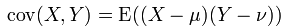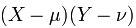其实，这种相乘的形式也有点类似于向量投影，即两个向量的内积。再远一点，联想到傅里叶变换里频谱系数的确定，要确定一个函数f(x)在某个频率w上的频谱，就是<f(x),cos(wt)>,< ,>表示向量内积，通俗的讲是将f(x)投影到cos(wt)上，要讲清傅里叶的本质需要另写一篇博文，这里提到这些只是觉得有益于对知识的相互理解。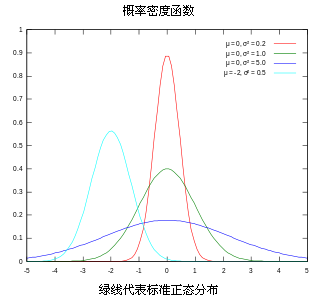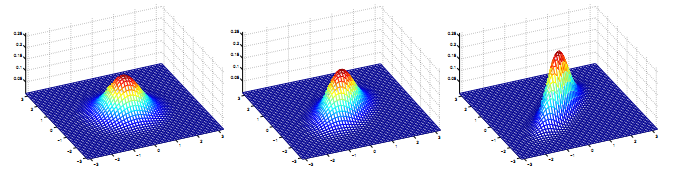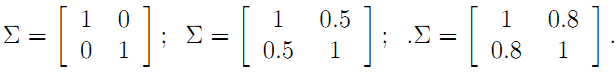-----------------------------------------------------------分割线---------------------------------------------------------------------

这部分每讲一个数学性的东西，接着就会有相应的例子和直观的分析帮助理解。

首先假设我们知道一个线性系统的状态差分方程为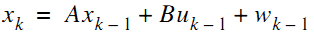看一个具体的匀加速运动的实例。

有一个匀加速运动的小车，它受到的合力为 ft , 由匀加速运动的位移和速度公式，能得到由 t-1 到 t 时刻的位移和速度变化公式：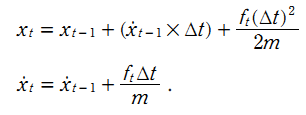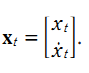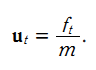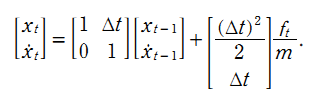貌似有了这个模型就能完全估计系统状态了，速度能计算出，位移也能计算出。那还要卡尔曼干嘛，问题是很多实际系统复杂到根本就建不了模。并且，即使你建立了较为准确的模型，只要你在某一步有误差，由递推公式，很可能不断将你的误差放大A倍（A就是那个状态转换矩阵），以至于最后得到的估计结果完全不能用了。回到最开始的那个笑话，如果那个完全凭预测的特种兵在某一步偏离了正确的路径，当他站在错误的路径上（而他自己以为是正确的）做下一步预测时，肯定走的路径也会错了，到最后越走越偏。

既然如此，我们就引进反馈。从概率论贝叶斯模型的观点来看前面预测的结果就是先验，测量出的结果就是后验。

测量值当然是由系统状态变量映射出来的，方程形式如下：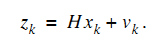同时对于匀加速模型，假设下车是匀加速远离我们，我们站在原点用超声波仪器测量小车离我们的距离。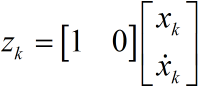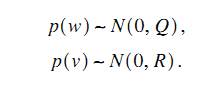对于小车匀加速运动的的模型，假设系统的噪声向量只存在速度分量上，且速度噪声的方差是一个常量0.01，位移分量上的系统噪声为0。测量值只有位移，它的协方差矩阵大小是1*1，就是测量噪声的方差本身。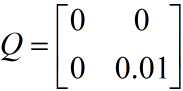Q中，叠加在速度上系统噪声方差为0.01，位移上的为0，它们间协方差为0，即噪声间没有关联。

理论预测（先验）有了，测量值（后验）也有了，那怎么根据这两者得到最优的估计值呢？首先想到的就是加权，或者称之为反馈。

我们认定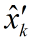是预测（先验）值，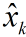是估计值，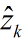为测量值的预测，在下面的推导中，请注意估计和预测两者的区别，不混为一谈。由一般的反馈思想我们得到估计值：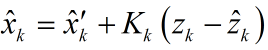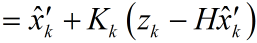现在的关键就是求取这个K。这时最小均方误差就起到了作用，顺便在这里回答为什么噪声必须服从高斯分布，在进行参数估计的时候，估计的一种标准叫最大似然估计，它的核心思想就是你手里的这些相互间独立的样本既然出现了，那就说明这些样本概率的乘积应该最大（概率大才出现嘛）。如果样本服从概率高斯分布，对他们的概率乘积取对数ln后，你会发现函数形式将会变成一个常数加上样本最小均方误差的形式。因此，看似直观上很容易理解的最小均方误差理论上来源就出于那里（详细过程还请自行谷歌，请原谅，什么都讲的话就显得这边文章没有主次了）。

先看估计值和真实值间误差的协方差矩阵，提醒一下协方差矩阵的对角线元素就是方差，求这个协方差矩阵，就是为了利用他的对角线元素的和计算得到均方差.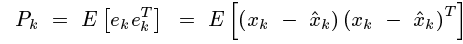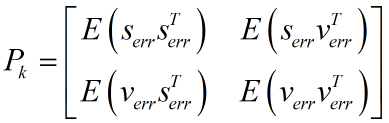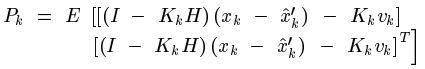(1)式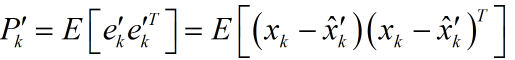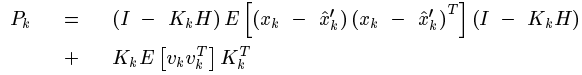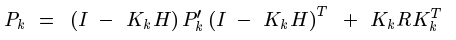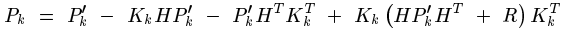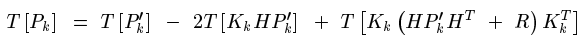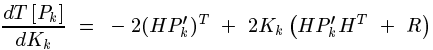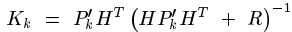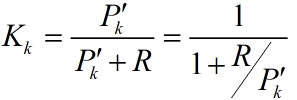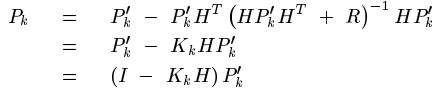请先注意到预测值的递推形式是：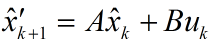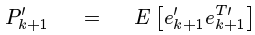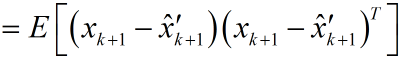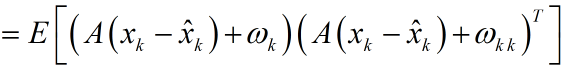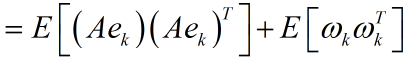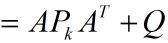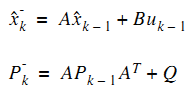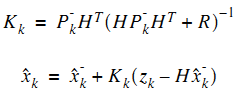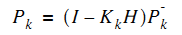（转载请注明作者和出处：http://blog.csdn.net/heyijia0327 未经允许请勿用于商业用途）

reference：

1.Greg Welch & Gary Bishop. << An Introduction to the Kalman Filter >>
2.Tony Lacey. << Tutorial:The Kalman Filter >>.

3.Ramsey Faragher. << Understanding the Basis of the Kalman Filter Via a Simple and Intuitive Derivation >>

4.highgear . 《授之以渔：卡尔曼滤波器...大泄蜜...》

5.很多概念定义来自维基百科

# 卡尔曼滤波 -- 从推导到应用(二)

1. clc
2. clear all
3. close all
4.
5. % 初始化参数
6. delta_t=0.1;   %采样时间
7. t=0:delta_t:5;
8. N = length(t); % 序列的长度
9. sz = [2,N];    % 信号需开辟的内存空间大小  2行*N列  2:为状态向量的维数n
10. g=10;          %加速度值
11. x=1/2*g*t.^2;      %实际真实位置
12. z = x + sqrt(10).*randn(1,N); % 测量时加入测量白噪声
13.
14. Q =[0 0;0 9e-1]; %假设建立的模型  噪声方差叠加在速度上 大小为n*n方阵 n=状态向量的维数
15. R = 10;    % 位置测量方差估计，可以改变它来看不同效果  m*m      m=z(i)的维数
16.
17. A=[1 delta_t;0 1];  % n*n
18. B=[1/2*delta_t^2;delta_t];
19. H=[1,0];            % m*n
20.
21. n=size(Q);  %n为一个1*2的向量  Q为方阵
22. m=size(R);
23.
24. % 分配空间
25. xhat=zeros(sz);       % x的后验估计
26. P=zeros(n);           % 后验方差估计  n*n
27. xhatminus=zeros(sz);  % x的先验估计
28. Pminus=zeros(n);      % n*n
29. K=zeros(n(1),m(1));   % Kalman增益  n*m
30. I=eye(n);
31.
32. % 估计的初始值都为默认的0，即P=[0 0;0 0],xhat=0
33. for k = 9:N           %假设车子已经运动9个delta_T了，我们才开始估计
34.     % 时间更新过程
35.     xhatminus(:,k) = A*xhat(:,k-1)+B*g;
36.     Pminus= A*P*A'+Q;
37.
38.     % 测量更新过程
39.     K = Pminus*H'*inv( H*Pminus*H'+R );
40.     xhat(:,k) = xhatminus(:,k)+K*(z(k)-H*xhatminus(:,k));
41.     P = (I-K*H)*Pminus;
42. end
43.
44. figure
45. plot(t,z);
46. hold on
47. plot(t,xhat(1,:),'r-')
48. plot(t,x(1,:),'g-');
49. legend('含有噪声的测量', '后验估计', '真值');
50. xlabel('Iteration');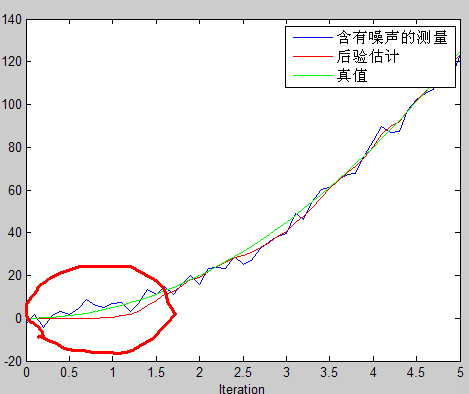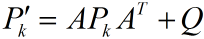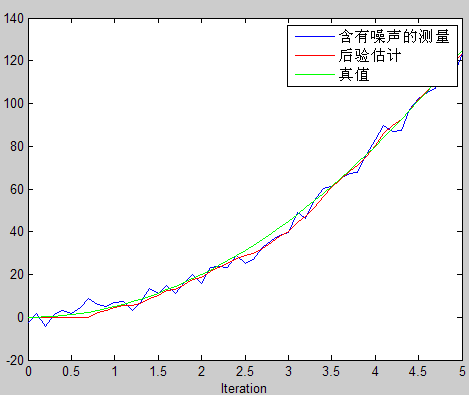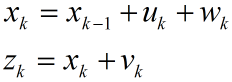1. clc
2. clear
3. close all
4.
5. % 初始化参数
6. delta_t=0.1;
7. t=0:delta_t:5;
8. g=10;%加速度值
9. n_iter = length(t); % 序列的长度
10. sz = [n_iter, 1]; % 信号需开辟的内存空间大小
11. x=1/2*g*t.^2;
12. x=x';
13. z = x + sqrt(10).*randn(sz); % 测量时加入测量白噪声
14.
15. Q = 0.9; % 过程激励噪声方差
16.          %注意Q值得改变  待会增大到2，看看效果。对比看效果时，修改代码不要改变z的值
17. R = 10; % 测量方差估计，可以改变它来看不同效果
18.
19. % 分配空间
20. xhat=zeros(sz);      % x的后验估计
21. P=zeros(sz);         % 后验方差估计
22. xhatminus=zeros(sz); % x的先验估计
23. Pminus=zeros(sz);    % 先验方差估计
24. K=zeros(sz);         % Kalman增益
25.
26. % 估计的初始值
27. xhat(1) = 0.0;
28. P = 1.0;
29. for k = 2:n_iter   %
30.     % 时间更新过程
31.     xhatminus(k) = xhat(k-1);
32.     Pminus(k) = P(k-1)+Q;
33.
34.     % 测量更新过程
35.     K(k) = Pminus(k)/( Pminus(k)+R );
36.     xhat(k) = xhatminus(k)+K(k)*(z(k)-xhatminus(k));
37.     P(k) = (1-K(k))*Pminus(k);
38. end
39.
40. figure
41. plot(t,z);
42. hold on
43. plot(t,xhat,'r-')
44. plot(t,x,'g-');
45. legend('含有噪声的测量', '后验估计', '真值');
46. xlabel('Iteration');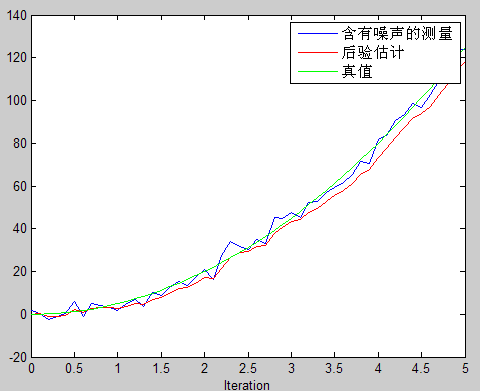图(a)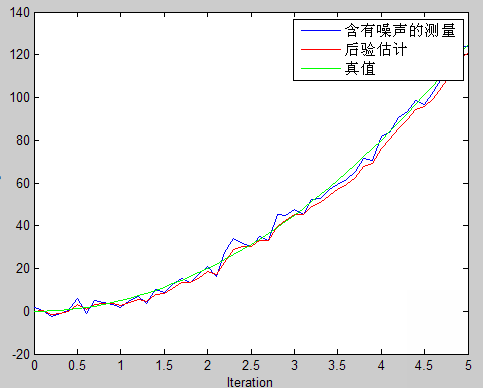图(b)

posted on 2018-06-25 13:56  AlanTu  阅读(3278)  评论(0编辑  收藏  举报